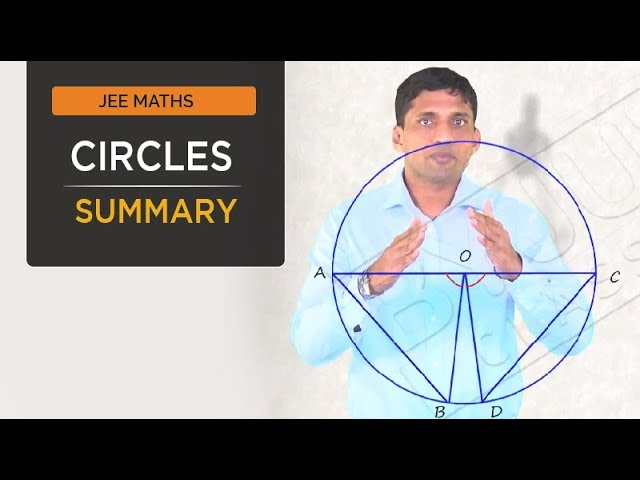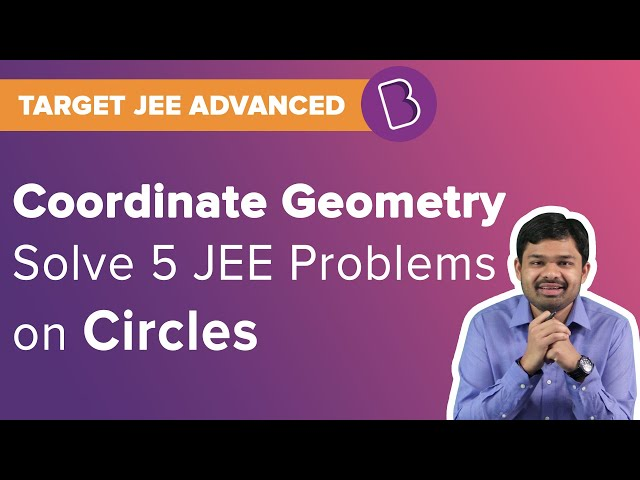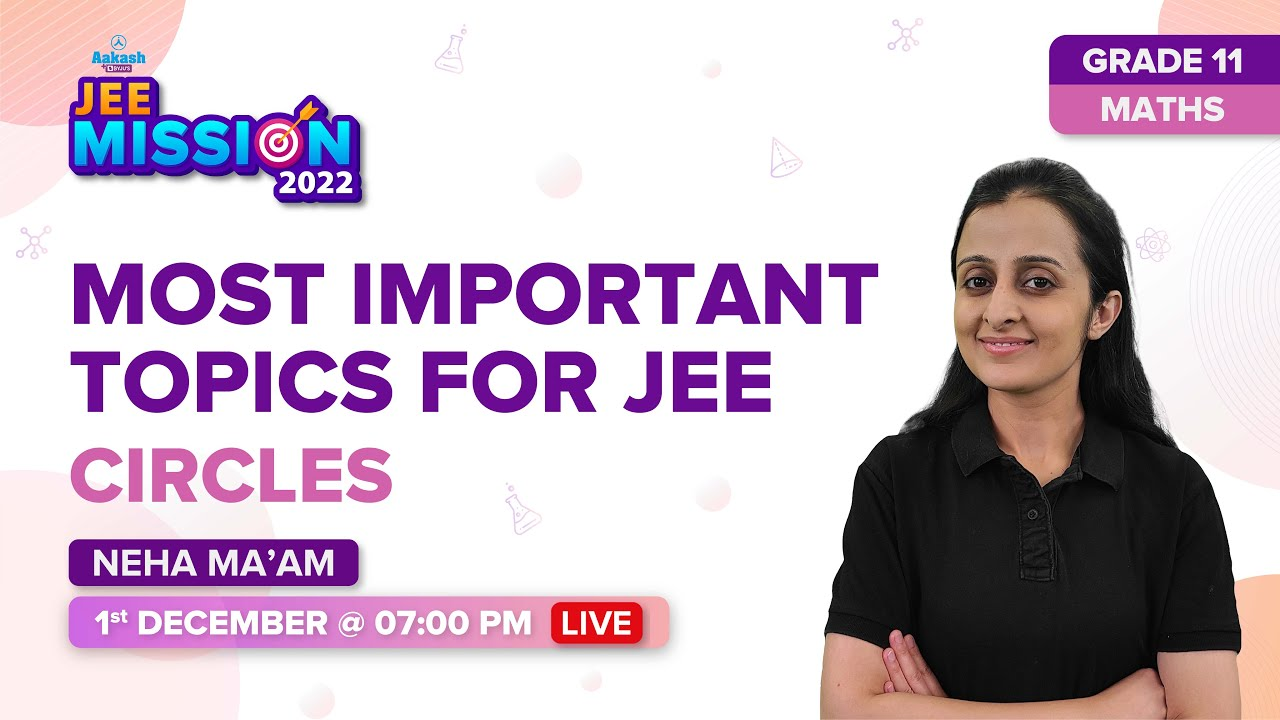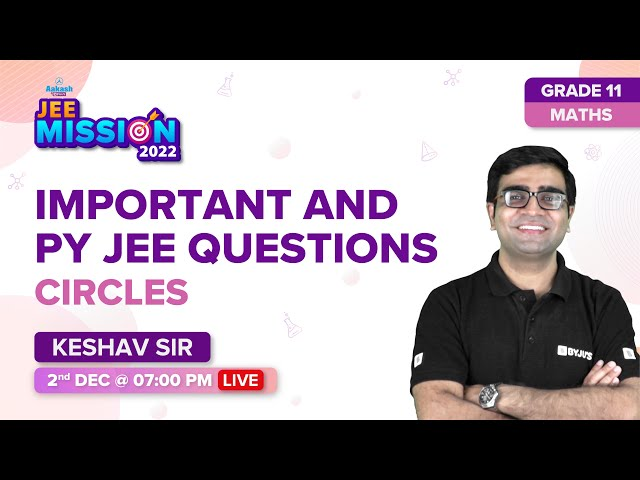Checkout JEE MAINS 2022 Question Paper Analysis : Checkout JEE MAINS 2022 Question Paper Analysis :

# JEE Advanced Maths Circles Previous Year Questions with Solutions

Students can find the JEE Advanced Maths circles previous year questions and solutions on this page. In the conic section, the topic circles have great importance. Students can expect questions from this topic. Revising previous year questions will help students to know about the exam pattern and difficulty levels of the exam. They can also download the solutions in PDF format for offline use.

Question 1: A circle S passes through the point (0, 1) and is orthogonal to the circles (x-1)2 + y2 = 16 and x2 + y2 = 1. Then

(a) radius of S is 8

(b) radius of S is 7

(c) centre of S is (-7, 1)

(d) centre of S is (-8, 1)

Solution:

Let the equation of the circles be

x2+y2+2gx+2fy+c = 0 ..(i)

It passes through (0, 1)

=> 1 + 2f + c = 0 …(ii)

Since circle (i) is orthogonal to (x-1)2 + y2 = 16

=> x2 + y2 – 2x – 15 = 0

and x2 + y2 – 1 = 0

2g×(-1) + 2f×0 = c-15

2g + c – 15 = 0 …(iii)

2g×0 + 2f×0 = c-1

=> c = 1 …(iv)

Solving (ii), (iii) and (iv)

=> c = 1, g = 7 and f = -1

The required circle is x2 + y2 + 14x – 2y + 1 = 0, with centre (-7, 1) and radius = 7.

Hence option b and c are correct.

Question 2: Let O be the centre of the circle x2 + y2 = r2, where r > √5/2 . Suppose PQ is a chord of this circle and the equation of the line passing through P and Q is 2x + 4y = 5. If the centre of the circumcircle of the triangle OPQ lies on the line x + 2y = 4, then the value of r is

(a) 1

(b) 4

(c) 6

(d) 2

Solution:

S1: x2 + y2 = r2 where r > √5/2

C1 = (0,0)

let S2: x2 + y2 + ax + by = 0

C2 = (-a/2, -b/2)

PQ: S1 -S2 = 0

PQ: ax + by + r2 = 0 ………………… (1)

Given PQ: 2x + 4y – 5 = 0 ………………… (2)

comparing equation (1) and (2)

(a/2) = (b/4) = (r2/-5)…………..…..(3)

Also, centre of S2 lies on x + 2y = 4

(-a/2) – b = 4 …(4)

From equation (3) and (4)

r = 2

Hence option d is the answer.

Question 3: The circle passing through the point (-1, 0) and touching the y-axis at (0, 2) also passes through the point

(a) (-3/2, 0)

(b) (-5/2, 2)

(c) (-3/2, 5/2)

(d) (-4, 0)

Solution:

Equation of circle passing through a point (x1, y1) and touching the straight line L, is given by

(x – x1)2 + (y – y1)2 + λL = 0

The circle is passing through the point (0, 2)

=> (x – 0)2 + (y – 2)2 + λL = 0

=> x2 + (y – 2)2 + λx = 0 …(i)

Circle passes through (-1, 0).

=> 1 + 4 – λ = 0

=> λ = 5

Put λ = 5 in (i)

x2 + (y – 2)2 + 5x = 0

=> x2 + y2 – 4y + 4 + 5x = 0

=> x2 + y2 + 5x – 4y + 4 = 0

Put y = 0

=> x = -4, x = -1

Check the options.

Hence option d is the answer.

Question 4: The number of common tangents to the circles x2 + y2 = 4 and x2 + y2 – 6x – 8y = 24 is

(a) 1

(b) 2

(c) 0

(d) 3

Solution:

Given circle x2 + y2 = 4 with centre C1(0, 0) and R1 = 2

Also x2 + y2 – 6x – 8y – 24 = 0 with centre C2(3, 4) and R2 = 7

Distance between centres = C1C2 = 5 = R2 – R1

So the circles touch internally and they can have just one common tangent at the point of contact.

Hence option a is the answer.

Question 5: The points of intersection of the line 4x – 3y – 10 = 0 and the circle x2 + y2 – 2x + 4y – 20 = 0 are

(a) (4, 2)

(b) (-2, -6)

(c) (2, 2)

(d) (-2, -4)

Solution:

Given equation of the line 4x – 3y – 10 = 0

=> x = (3y+10)/4 …(i)

Equation of the circle x2 + y2 – 2x + 4y – 20 = 0 ..(ii)

Put (i) in (ii)

=> [(3y+10)2/42 ] + y2 – 2(3y+10)/4 + 4y – 20 = 0

=> y2 + 4y – 12 = 0

=> y = 2, -6

Put y in (i)

=> x = 4, -2

So the points are (4, 2) and (-2, -6).

Hence option a and b are correct.

Question 6: If the tangent at (1,7) to the curve x2 = y-6 touches the circle x2 + y2 + 16x + 12y + c = 0, then the value of c is

(a) 185

(b) 85

(c) 195

(d) 95

Solution:

Given curve is x2 = y – 6

Differentiate w.r.t.x

2x = dy/dx

(dy/dx)(1,7) = 2

Equation of tangent at (1, 7) to x2 = y – 6 is

y-y1 = m(x-x1)

Here m = 2

(y-7) = 2 (x – 1)

2x – y + 5 = 0

The perpendicular from the centre (-8, -6) to 2x – y + 5 = 0 is equal to radius of circle.

So |(-16+6+5)/√5 | = √(64 + 36 – c)

=> 5 = 100 – c

=> c = 95

Hence option d is the answer.

Question 7: The circle passing through the intersection of the circles, x2 + y2 – 6x = 0 and x2 + y2 – 4xy = 0, having its centre on the line, 2x – 3y + 12 = 0, also passes through the point

(a) (-1, 3)

(b) (-3, 6)

(c) (-3, 1)

(d) (1, -3)

Solution:

Let the family of circles be S1 + λS2 = 0

x2 + y2 – 6x + λ(x2 + y2 – 4y) = 0

=> (1+λ)x2 + (1+λ)y2 – 6x – 4λy = 0 …(i)

Centre (-g, -f) = (3/(1+λ), 2λ/(1+λ))

Centre lies on 2x – 3y + 12 = 0

Then (6/(λ+1)) – (6λ/(λ+1)) + 12 = 0

=> λ = -3

Equation of circle (i)

-2x2 – 2y2 – 6x + 12y = 0

=> x2 + y2 + 3x – 6y = 0

Check options.

(-3, 6) satisfy equation (ii).

Hence option b is the answer.

Question 8: Let the tangents drawn from the origin to the circle, x2 + y2 – 8x – 4y + 16 = 0 touch it at points A and B. Then (AB)2 is equal to

(a) 52/5

(b) 56/5

(c) 64/5

(d) 32/5

Solution:

Length of tangent, L = √S1 = √16 = 4

R = √(16 + 4 – 16)

= 2

Length of chord ofcontact = 2LR/√(L2+R2)

= 16/√20

Square of length of chord of contact = 64/5

Hence option c is the answer.

Question 9: The centre of the circle inscribed in the square formed by the lines x2 – 8x + 12 = 0 and y2 – 14y + 45 = 0

(a) (4, 7)

(b) (7, 4)

(c) (9, 4)

(d) (4, 9)

Solution:

The centre is given by the intersection of the diagonals (the mid-point of a diagonal).

x2 – 8x + 12 = 0

=> (x – 6)(x-2) = 0

=> x = 6 , 2

y2 – 14y + 45 = 0

=> (y – 5)(y-9) = 0

=> y = 5, 9

So A(2, 5), B(2, 9), C(6, 9), D(6, 5) form the square.

Therefore the centre of circle inscribed in square will be

((2+6)/2, (5+9)/2) = (4, 7)

Hence option a is the answer.

Question 10: If the tangent at the point P on the circle is x2 + y2 + 6x + 6y = 2 meets a straight line 5x – 2y + 6 = 0 at a point Q on the y-axis, then the length of PQ is

(a) 4

(b) 5

(c) 2√5

(d) 3√5

Solution:

Given that the line 5x – 2y + 6 = 0 is intersected by tangent at P to the circle x2 + y2 + 6x + 6y – 2 = 0 on y ais at Q.

On y axis, x = 0

=> 2y = 6

=> y = 3

So Q is (0, 3)

Tangent passes throught (0, 3).

PQ = length of tangent to the circle from (0, 3)

= √(0+9+0+18-2)

= 5

Hence option b is the answer.

Recommended videos

## JEE Math Preparation Tips – Summary of Circles## Circles – JEE Advanced Solved Questions## Circles- Important Topics for JEE## Circles – Important and Previous Year JEE Questions